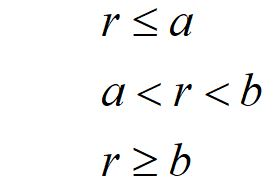﻿ Using Gauss's law

# Using Gauss's law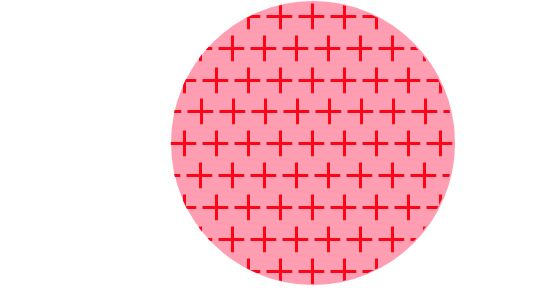## We can use Gauss's law to calculate the electric field of a sphere with a spherically symmetric charge. For simplicity, we will use a constant charge density.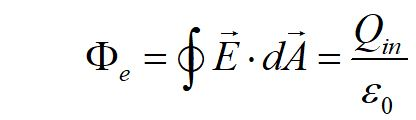## We begin with the general Gauss's law, relating electric flux to a closed integral, to the enclosed charge.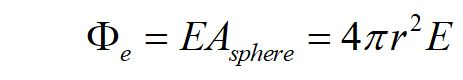## To find the electric field everywhere outside the sphere, We integrate over the outer surface area of the sphere.## Using the relationship that the total flux equals the charge enclosed over the permittivity constant, We can solve to the E field. The field points radially away from the spherically symmetric charge density.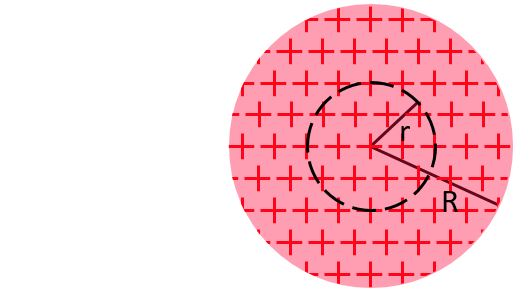## We can use a similar method to find the electric field inside a uniform sphere of charge.## We use the definition of a constant volume charge density to write the equation for the charge enclosed inside a volume of radius r, inside the sphere.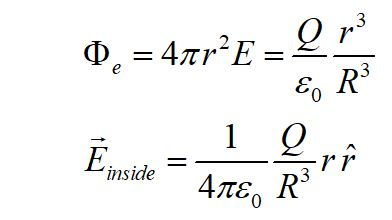## Inserting this Qin, we can write the equation for the flux inside the sphere, and for the electric field inside the sphere.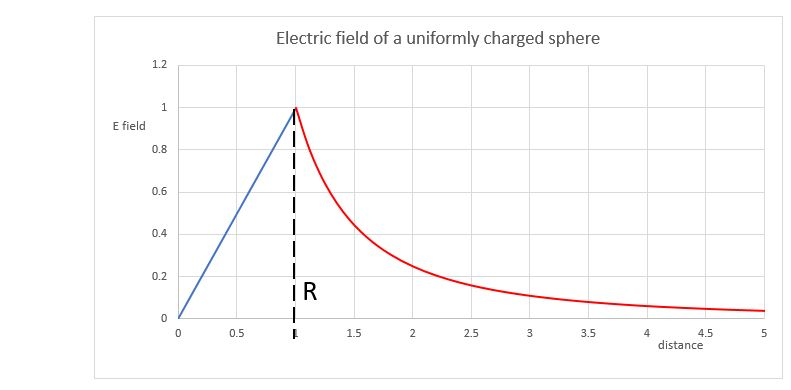## A conductor in electrostatic equilibrium has no current inside, that is, by definition, there are no charges moving. This means that the electric field inside the conductor must be zero, otherwise the electric field would cause charge carriers to move.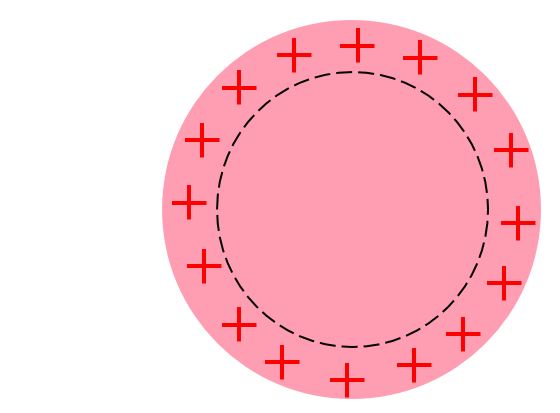## A hollow conducting sphere has an inner edge of radius a and an outer edge of radius b. The sphere has a charge of +2Q, and a point charge of +Q sits at the center of the sphere.## Find the electric field for the regions: